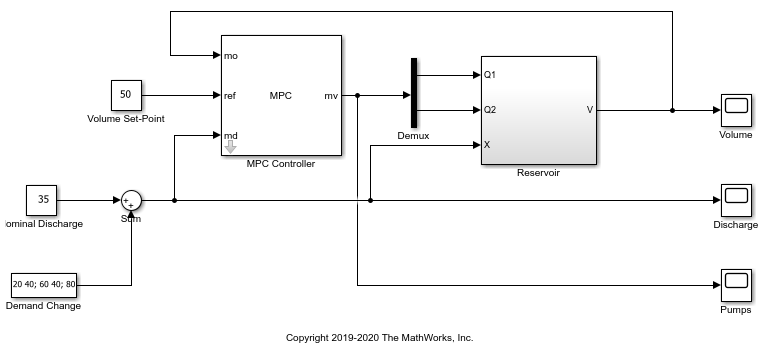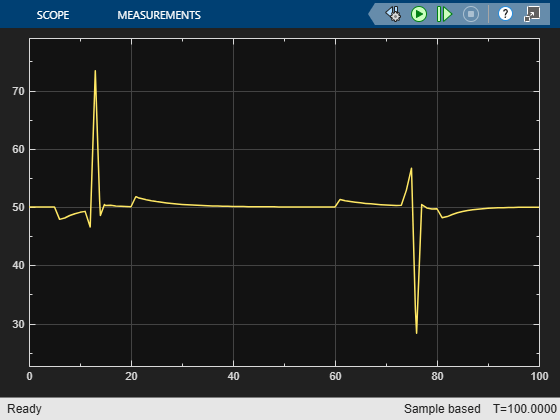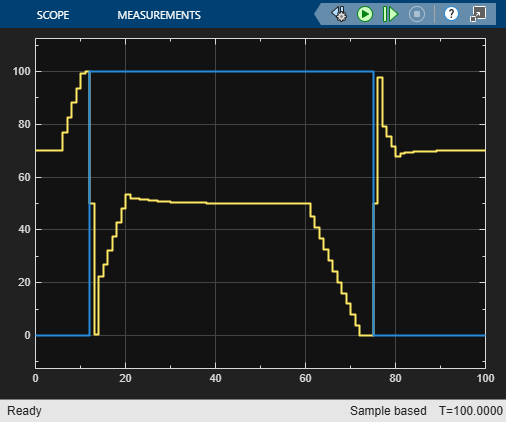# Surge Tank Control Using Discrete Control Set MPC

This example shows how to use a linear MPC controller with both continuous- and discrete-set control actions to control the level of a surge tank in Simulink®.

### Overview

Many petrochemical processes include surge capacity to insulate downstream operations from upsets in upstream flows. This example considers a liquid surge tank supplied by two pumps.

• Pump 1 is variable-speed and can deliver a flow rate between 0 and 100 L/min. The rate of change of the flow rate is limited to 50 L/min per minute.

• Pump 2 is on-off and delivers 100 L/min when it is on.

This system also has a continuously adjustable valve that regulates the tank discharge to accommodate a downstream process.

```mdl = 'ReservoirMPC'; open_system(mdl) ```The control objective is to use the two pumps (manipulated variables) to maintain the tank volume at its setpoint when the discharge valve introduces a disturbance to the surge tank (measured disturbance).

When downstream demand is constant, pump 1 controls the surge tank level, ideally keeping it near 50%. When a disturbance occurs, both pumps can go into action to maintain the tank volume between 25% and 75% during the transient time. When downstream demand is high, however, Pump 2 must turn on.

In summary, the MPC controller manages the two pumps such that:

• The tank level stays near 50% when demand is constant.

• The tank level stays within the ideal range when Pump 2 turns on and off. Rapid Pump 2 on-off cycling is undesirable.

### Create Linear Plant from Nonlinear Surge Tank Model

In the Simulink model, the surge tank model is in the Reservoir subsystem. It implements the following equations.

• `dV/dt = Qin - Qout`, where `V` is the tank volume between 0 and 100 (percent)

• `Qin = Q1 + Q2`, the sum of the two pump flow rates (L/min)

• `Qout = 0.2*sqrt(2)*X*sqrt(V)`, the discharge rate (L/min)

• `X`, the discharge valve, which can open between 0 and 100 (percent)

The plant starts running at its nominal steady-state operating point: pump 1 runs at 70 L/min, pump 2 is off, the discharge valve is at 35%, and the tank volume is at 50%. Create a linear model of the tank at this operating point. This model has three inputs: the manipulated variables `Q1` and `Q2`, and the measured disturbance|X|.

```plant = ss(-0.7,[1 1 -2],1,[0 0 0]); plant = setmpcsignals(plant,'MV',[1 2],'MD',3); ```

### Design MPC Controller with Continuous and Discrete Control Actions

Create a linear MPC controller with a sample time of one second, default prediction and control horizons, and default cost function weights.

```Ts = 1; mpcobj = mpc(plant,Ts); ```
```-->"PredictionHorizon" is empty. Assuming default 10. -->"ControlHorizon" is empty. Assuming default 2. -->"Weights.ManipulatedVariables" is empty. Assuming default 0.00000. -->"Weights.ManipulatedVariablesRate" is empty. Assuming default 0.10000. -->"Weights.OutputVariables" is empty. Assuming default 1.00000. ```

Set the nominal values for the controller to match the steady-state operating point.

```mpcobj.Model.Nominal.U = [70; 0; 35]; mpcobj.Model.Nominal.Y = 50; mpcobj.Model.Nominal.X = 50; mpcobj.Model.Nominal.DX = 0; ```

Choose the `MVRate` weights such that the controller adjusts pump 1 in preference to pump 2. That is, the controller penalizes pump 2 adjustments less than pump 1 adjustments.

```mpcobj.Weights.MVrate = [0.1 0.2]; ```

Specify safety bounds on the continuous input and output signals.

```mpcobj.OV.Min = 0; mpcobj.OV.Max = 100; mpcobj.MV(1).Min = 0; mpcobj.MV(1).Max = 100; mpcobj.MV(1).RateMin = -50; mpcobj.MV(1).RateMax = 50; ```

Since pump 2 has two discrete settings (0 and 100 L/min), specify a discrete set in the `Type` property of this controller. Depending on your application, the `Type` property can also be `binary` or `integer`.

```mpcobj.MV(2).Type = [0 100]; ```

### Simulate Closed-Loop Response with Discharge Disturbance

The simulation begins at the nominal condition. At t = 5, the discharge rate ramps up until pump 1 is at its full capacity and pump 2 must turn on. Pump 2 turns on at t = 12. Pump 1 must then decrease as rapidly as possible to keep the level in bounds, then establish a new steady-state operating point. At t = 60, the discharge begins to ramp down to the nominal state. The MPC controller keeps the volume within the ideal range, and pump 2 does not exhibit rapid cycling.

```sim(mdl) open_system([mdl '/Volume']) ```
```-->Converting model to discrete time. -->Assuming output disturbance added to measured output #1 is integrated white noise. -->"Model.Noise" is empty. Assuming white noise on each measured output. ans = Simulink.SimulationOutput: tout: [106x1 double] SimulationMetadata: [1x1 Simulink.SimulationMetadata] ErrorMessage: [0x0 char] ```The following figure shows the flow rates from two pumps.

```open_system([mdl '/Pumps']) ````As expected, the MPC controller turns pump 2 on and off.`
```bdclose(mdl) % close simulink model. ```